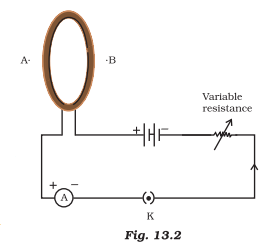# A circular loop placed in a plane perpendicular to the plane of paper carries a current when the key is ON. The current as seen from points A and B (in the plane of the paper and on the axis of the coil) is anti-clockwise and clockwise respectively. The magnetic field lines point from B to A. The N-pole of the resultant magnet is on the face close to (a) A (b) B (c) A if the current is small, and B if the current is large (d) B if the current is small and A if the current is large(a) A

### Explanation

The direction of the magnetic field will be from south to north pole. Point A is showing North Pole because field lines are pointing from B to A. A circular loop placed in a plane perpendicular to the plane of paper, carries a current when the key is ON. The current, as seen from points A and B(in the plane of the paper and on the axis of the coil) is anticlockwise and clockwise respectively. The magnetic field lines point from B to A.(1)(0)